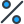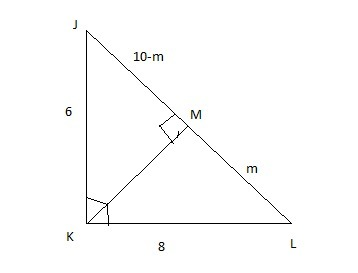# The figure shows three right triangles. Triangles JKM, KLM, and JLK are similar. Theorem: If two triangles are similar, the corresponding sides are in proportion. Figure shows triangle JKL with right angle at K. Segment JK is 6 and segment KL is 8. Point M is on segment JL and angles KMJ and KML are right angles. Using the given theorem, which two statements help to prove that if segment JL is x, then x2 = 100? Segment JL • segment JM = 64 Segment JL • segment ML = 48 Segment JL • segment JM = 48 Segment JL • segment ML = 36 Segment JL • segment JM = 64 Segment JL • segment ML = 36 Segment JL • segment JM = 36 Segment JL • segment ML = 64

•Subject:

Mathematics
• Author:

elianna
• Created:

7 months ago

(Segment JL)(Segment ML)=10(6.4)=64 units

Step-by-step explanation:

In the given information, triangle JKL with right angle at K. Segment JK is 6 and segment KL is 8. Point M is on segment JL and angles KMJ and KML are right angles.

we have to choose the correct option.

In order to choose we have to find the segment ML

Let ML=x therefore JM=10-m

In triangle JMK, by Pythagoras theorem

JK^{2}=JM^{2}+MK^{2}\\  36=(10-m)^2+KM^2\\KM^2=36-(10-m)^2

In triangle KML

KL^{2}=KM^{2}+ML^{2}\\  64=m^2+KM^2\\KM^2=64-m^2

From above two equations we get

36-(10-m)^2=64-m^2

⇒ 64-m^2=36-(10-m)^2

⇒ 20m=\frac{128}{20}

⇒ m=6.4 units

(Segment JL)(Segment ML)=10(6.4)=64 units

Hence, last option is correctSegment JL × segment JM = 36

Segment JL × segment LM = 64

Step-by-step explanation:

I'm smart.

. The two statements that help to prove that if segment JL is x, then x2 = 100 are: Segment JL • segment JM = 64 and Segment JL • segment ML = 48. These two statements help to prove that if segment JL is x, then x2 = 100 because if the corresponding sides of the similar triangles are in proportion, then the ratio of the lengths of the sides is equal to the square of the ratio of the segments. Since the ratio of the segments JK : KL is 6 : 8, this means that the ratio of any segment of triangle JKL to the corresponding segment of triangle KLM is equal to the square of the ratio of 6 : 8, or 36 : 64. So if segment JL is x, then the ratio of x to the corresponding segment (JM or ML) is equal to 36 : 64 and x2 = 100.

Don't worry! There are several alternative approaches you can try to resolve your query. Here are some tips to help you find answers in different ways:

1. Reframe your question: Sometimes, the way you phrase your question can limit your search results. Try rephrasing it using different keywords or providing more context to get better results.
2. Utilize social media: Post your question on social media platforms, particularly those focused on professional or specialized topics. Twitter, LinkedIn, and Facebook groups can connect you with individuals who may have relevant expertise or experiences to share.
3. Consult subject matter experts: Reach out to experts in the field related to your question. Many professionals are willing to help and share their knowledge. You can connect with them through email, LinkedIn messages, or by attending relevant conferences or events.
4. Use our website to find your question through the search box above, or you can sign up to ask your question for our big educational community. Our experts will review your question, and you will get a quick and quality answer.
5. Collaborate with others: If your question is related to a specific project or problem, consider collaborating with others who might have complementary skills or knowledge. Teamwork can lead to innovative solutions and shared insights.

Remember, the process of finding answers often involves persistence, creativity, and an open mind. By exploring various resources, reaching out to others, and being proactive in your search, you increase your chances of finding the information you need. Happy quest for knowledge!